# Looking Up Values in Tables

Problem

You're using tabular data in calculations and you need an easy way to look up values in the table for use in other calculations.

Solution

Use the built-in functions Lookup, VLookup, HLookup, Match, or Index.

Discussion

Excel provides five functions to help you access data in tabular form. These functions come in handy when performing calculations that require looking up data from standard tables. For example, you can develop a spreadsheet to perform thermodynamic calculations and include standard steam tables in this spreadsheet. This way, instead of manually looking up fluid properties to use in your calculations, you can automatically include that data via the lookup functions discussed here.

LOOKUP

The syntax for LOOKUP is =LOOKUP(lookup value, lookup vector, result vector). Lookup finds the value in result vector corresponding to the position of the value in lookup vector that matches lookup value. The lookup vector must be sorted in ascending order (see Recipe 3.9). Also, the lookup and result vectors may be row or column ranges.

You can use text or a number as the lookup value; the function just compares the lookup value to the data stored in the lookup vector to find a match. This is an important caveat with this function (and the others discussed here), as no interpolation is performed. The function looks for an exact match and if it does not find one, it returns the value in the result vector corresponding to the value in the lookup vector that is closest to but less than the lookup value. (Take a look at the recipes in Chapter 8 to learn how to interpolate data in Excel.)

Figure 3-24 illustrates a typical application of LOOKUP. In this example, we have a simple table of data that presents design factors, say for ship structural design, as a function of length of the ship under consideration, the ship's hull material, and the units used. (Don't bother looking up this table of data in a marine design handbookit doesn't exist. I just made it up for illustration purposes.)

Let's say we have a design formula, or better yet a whole series of calculations that must be performed, that requires the design factor as input. Including the design factor table in the spreadsheet allows you to conveniently select the factor by specifying the length, material type, and units, rather than looking up the data in a handbook and entering it manually. This is especially handy if you distribute your spreadsheet to someone else.

The three values in cells F14 through F16 are user input values corresponding to the given length, units, and material, respectively. The design factor corresponding to this given data is contained in cell F17. The formula in cell F17 that actually performs the lookup is:

``` =LOOKUP(F14, IF(F15="ft",D8:D12,E8:E12), IF(F16="Steel", F8:F12,G8:G12))
```

This formula looks more complicated than it is, due to the nested IF functions.

Figure 3-24. LOOKUP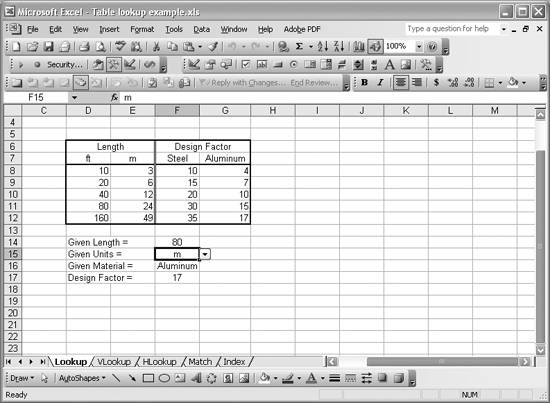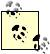IF functions are like conditional statements in traditional programming languages. They are quite handy for use in spreadsheets. The syntax for the IF function is =IF(logical expression, value if true, value if false). The logical expression can be any expression that evaluates to true or false. You can use cell references in a logical expression along with logical operators such as >, <, and =. There are also a few other logical functions (AND, OR, and NOT) that you can use to construct more elaborate logical statements like =IF(AND(C5>F9, G9=2), ... ) or =IF(AND(OR(C5>F9, G9=2), NOT(C11)), ...,). value if true is what will be used if the logical expression evaluates to true, whereas value if false will be used if the logical expression evaluates to false. Either of these values may be hardcoded numbers or text, cell references, or nested functions (for example, you can construct nested conditional statements by including another IF function as value if true or value if false, and so on.

Basically, the given length, cell F14, is used as the value to look up in the lookup vector, but the lookup vector itself depends on which units are selected. An IF function is used to test the given units and return the correct lookup vector. Another IF function is used to test the given material and return the correct result vector. The design factor is extracted from the result vector on the row corresponding to the row containing the given lookup value (the given length, in this case).If you take a close look at Figure 3-24, you will notice a little down arrow button next to selected cell, F15, corresponding to the given units. Pressing this button will display a listbox allowing you to select which units to enter (ft or m) instead of manually typing the units. I used the same technique for the given material input cell. Using drop-down selection lists in this way is a great way to control the input value of a cell, being sure the value entered is a valid value. This provides a more robust spreadsheet, especially when the spreadsheet is distributed to other users. To actually get the cell to display a list of valid data, use Excel's Data Validation feature (see Recipe 1.15). The Allow field shown in Figure 1-21 includes a List option. When you select a List in the Allow field, a Source field will be displayed, allowing you to select a cell range containing the data to show in the list. For cell F15 in Figure 3-24, I set the source range to D7:E7, which includes the two valid options, ft and m.

VLOOKUP

The syntax for VLOOKUP is =VLOOKUP(lookup value, table, column, match flag). VLOOKUP searches for the given lookup value in the first column of the range of cells given by table and returns the value on the corresponding row in the given column. match flag is optional. If it is set to TRue or is omitted, then VLOOKUP will find an exact match or a close match. In this case, the first column in the given table should be sorted in ascending order (see Recipe 3.9). If an exact match is not found, the function returns the value in the given column corresponding to the value in the first column that is closest to but less than the lookup value. If match flag is false, VLOOKUP will look only for exact matches. If one is not found, the function returns #N/A, indicating the absence of an exact match.

Figure 3-25 shows a spreadsheet containing a table of physical properties for water. The first column of the table contains temperatures ranging from 10 to 90°C. Typical calculations would involve looking up physical properties in such a table for use in other calculations.

Physical properties of water for a given temperature can be retrieved from this table using VLOOKUP. In Figure 3-25, cell E17 contains the given temperature for which properties are retrieved. In this case, density, internal energy, enthalpy, entropy, specific heats, sound speed, and viscosity corresponding to the given temperature are shown in cells E18 through E25. Each of these cells contains a formula that utilizes VLOOKUP. For example, cell E18 contains the formula =VLOOKUP(\$E\$17,\$C\$4:\$K\$12,2). The value to be looked up in the first column of the data table is stored in cell E17; this is the given temperature. The data table itself is stored in the cell range C4:K12. The last parameter in this formula indicates the column in the data table from which to retrieve data corresponding to the given temperature. Column 2 in this case is the density of water. The remaining cells contain similar formulas. The only differences are the last parameter in VLOOKUP (i.e., the column index from which to retrieve the desired value).

Figure 3-25. VLOOKUP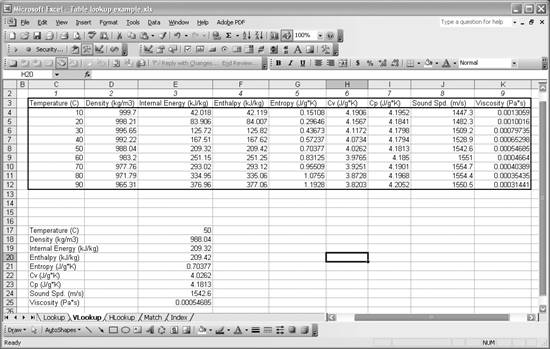HLOOKUP

HLOOKUP is very similar to VLOOKUP; however, HLOOKUP uses the first row of data in a given cell range as the lookup array. The syntax for HLOOKUP is = HLOOKUP(lookup value, table, row, match flag). The only difference between this syntax and that of VLOOKUP is the third parameter; HLOOKUP requires a row number to be used to retrieve the result.

Figure 3-26 contains the same data shown in Figure 3-25; however, in this new example the data has been transposed. Now, temperatures are on the first row instead of in the first column.It's easy to transpose data in Excel. Select the range of cells you want to transpose and copy it, using Ctrl-C or Edit [Image_Link]/images/U2192.jpg border=0> Copy. Select a new cell where you want to paste the data and select Edit [Image_Link]/images/U2192.jpg border=0> Paste Special ....In the Paste Special dialog box, check the Transpose box and press the OK button.

Physical properties of water for a given temperature can be retrived from this table using HLOOKUP. For example, cell D17 extracts the density corresponding to the temperature given in cell D16. The formula in cell D17 is =HLOOKUP(\$D\$16,\$D\$4:\$L\$12,2). The formulas for the other properties in cells D18 through D24 are very similar; only the result row number changes.

Figure 3-26. HLOOKUP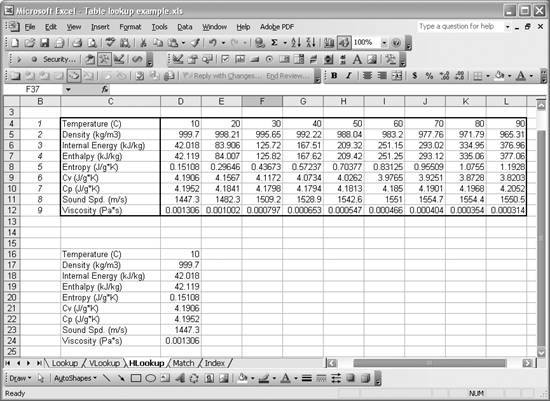MATCH

MATCH returns the position of an item in an array. For example, in the spreadsheet shown in Figure 3-27 we could use MATCH to return the position of the cell containing the text Sound Spd. (m/s) in the array of cells C4 to C12. In this case, the position returned would be 8. The syntax for MATCH is =MATCH(lookup value, lookup array, match type). Here, lookup value is the value to be looked up in the array lookup array. The last parameter, match type, tells MATCH how you want to determine a match. If match type is 0, then MATCH looks for an exact match. If match type is -1, then Match returns the position of the smallest value greater than or equal to lookup value. If match type is 1, then MATCH returns the position of the largest value less than or equal to lookup value. Remember, MATCH returns the position of the matched value, not the value itself.

The spreadsheet in Figure 3-27 shows how you might use MATCH. In this case, we have the same data table as in the HLOOKUP example. This time, however, in cell D18 we have this formula: =HLOOKUP(D17,D4:L12,MATCH(C18,C5:C12,0)+1). This time I nested a call to MATCH as the third parameter in HLOOKUP. In the previous example, I specified a row in which to look up the result. In this case, I use MATCH to find the row corresponding to the description of the property selected in cell C18. Cell C18 contains a drop-down listbox that allows you to select any of the properties in the table except the temperature property. I used the same data validation technique described earlier in this recipe to create the selection list in this example.

Figure 3-27. MATCH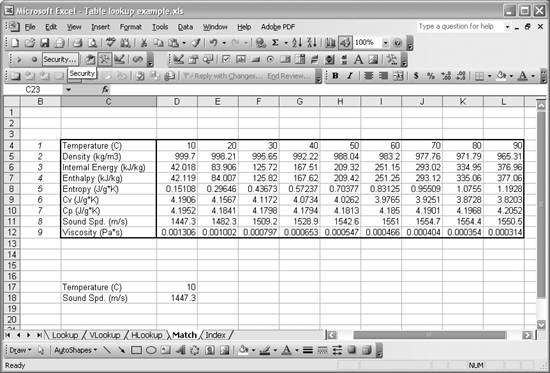The call to MATCH in the call to HLOOKUP returns the position of the selected description in the list of descriptions contained in cells C5 to C12. To get the proper row number for the table in the cell range C4:L12, you have to add 1 to the value returned by MATCH since the number of rows in the description cell list is shifted by 1 from the data table.

INDEX

INDEX is useful for extracting a value from a table (cell range) given the position of the value in the table. The syntax for INDEX is =INDEX(cell reference, row, column). INDEX will return the value in the cell at position row and column in the range specified by cell reference. This is like extracting the value of an element in a matrix given its row and column position.

Figure 3-28 shows a spreadsheet containing a set of data resembling a 4x3 matrix.

Cell D10 contains the value of element [2,2] extracted using INDEX. The formula in cell D10 is =INDEX(D4:F7,2,2).

If you want to extract an entire row, set the column to 0 in the call to INDEX. Likewise, to extract an entire column, set the row to 0. To extract an entire row or column at a time, you need to use array formulas. These are nothing more than formulas that act over a range of cells (instead of over a single cell, like the formulas I've discussed so far).

Figure 3-28. INDEX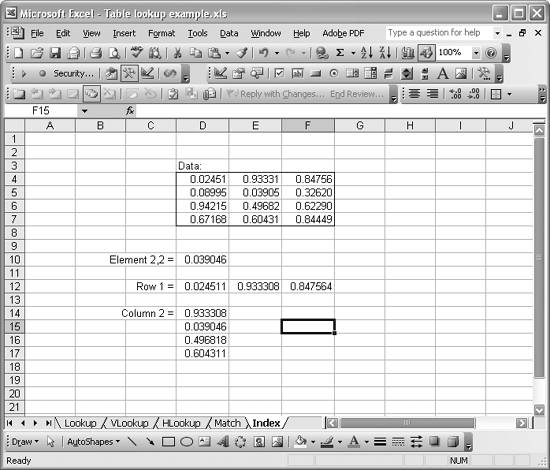To extract the first row as shown in Figure 3-28, I first selected the destination cell range D12:F12 by clicking and dragging the mouse. Then I pressed the equals sign to begin writing the formula: =INDEX(D4:F7,1,0). Notice that I set the column to 0, indicating that I want an entire row. To enter this formula, which is now an array formula, press Ctrl-Shift-Enter instead of the usual Enter. This tells Excel you're entering an array formula, and it will fill all the selected cells with results of the formula you entered.Array formulas are shown in the formula bar with braces around them, like {=INDEX(D4:F7,1,0)}. I show you how to use array formulas in other recipes throughout this book. Take a look at Recipe 5.2 and Recipe 7.11 for some examples.

To extract the entire second column as shown in Figure 3-28, select the range D14:D17 and Ctrl-Shift-Enter the formula =INDEX(D4:F7,0,2). In this case, the row is set to 0, indicating that you want to extract an entire column.

INDEX is handy when working with matrices or matrix-like data and calculations. See Chapter 7 for more recipes dealing with matrix calculations.

In this recipe, I used and briefly discussed Excel's IF function. While its use is fairly straightforward, you can read more about it and other logical functions in Excel's online help. Look for the topic "Logical Functions."Excel Scientific and Engineering Cookbook (Cookbooks (OReilly))
ISBN: 0596008791
EAN: 2147483647
Year: N/A
Pages: 206
Authors: David M Bourg